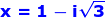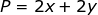Showing posts with label Algebra. Show all posts
Showing posts with label Algebra. Show all posts

## Saturday, December 6, 2014

### Theory of Equations, 8

Category: Algebra

"Published in Newark, California, USA"

Find the equation of a polynomial if the roots are 3, 1, and -2

Solution:

If one of the root of the equation is given which is -2 , then we need to get its conjugate because we want to eliminate the radical sign in the given equation. The conjugate of -2 is 2 . Hence, the equation or a factor from the product of a root and its conjugate is

Therefore, the equation of a polynomial is

## Friday, December 5, 2014

### Theory of Equations, 7

Category: Algebra

"Published in Newark, California, USA"

Find the remaining roots of the equation

if 1 - i2 is a root.

Solution:

If one of the root of the equation is given which is 1 - i2 , then we need to get its conjugate because we want to eliminate the imaginary number and the radical sign in the given equation. The conjugate of 1 - i2 is 1 + i2 . Hence, the equation or a factor from the product of a root and its conjugate is

In order to get the other factor for the given equation, let's divide the given equation with the above equation, we have

Since there's no remainder in the division, then the other factor of the given equation is x² + 2x - 3.

Let's factor the other factor of the given equation as follows

If you equate each factor to zero, then the values of x are -3 and 1.

Therefore, the other roots of the given equation are -3 and 1.

## Thursday, December 4, 2014

### Theory of Equations, 6

Category: Algebra

"Published in Newark, California, USA"

Find the remaining roots of the equation

if 2 + 5 is a root.

Solution:

If one of the root of the equation is given which is 2 + 5 , then we need to get its conjugate because we want to eliminate the radical sign in the given equation. The conjugate of 2 + 5 is 2 - 5. Hence, the equation or a factor from the product of a root and its conjugate is

In order to get the other factor for the given equation, let's divide the given equation with the above equation, we have

Since there's no remainder in the division, then the other factor of the given equation is 2x + 3.

Therefore, the other root of the given equation is.

## Wednesday, December 3, 2014

### Theory of Equations, 5

Category: Algebra

"Published in Newark, California, USA"

Find the remaining roots of the equation

if -2 is a root.

Solution:

If one of the root of the equation is given which is -2, then we need to get its conjugate because we want to eliminate the radical sign in the given equation. The conjugate of -2 is 2. Hence, the equation or a factor from the product of a root and its conjugate is

In order to get the other factor for the given equation, let's divide the given equation with the above equation, we have

Since there's no remainder in the division, then the other factor of the given equation is x² - 2x + 4.

By using the completing the square method, we can solve for the other roots of the given equation as follows

Therefore, the other roots of the given equation areand.

## Tuesday, December 2, 2014

### Theory of Equations, 4

Category: Algebra

"Published in Newark, California, USA"

Find the remaining roots of the equation

if 5 + i is a root.

Solution:

If one of the root of the equation is given which is 5 + i, then we need to get its conjugate because we want to eliminate the imaginary number in the given equation. The conjugate of 5 + i is 5 - i. Hence, the equation or a factor from the product of a root and its conjugate is

In order to get the other factor for the given equation, let's divide the given equation with the above equation, we have

Since there's no remainder in the division, then the other factor of the given equation is x + 2.

Therefore, the other root of the given equation is - 2.

## Monday, December 1, 2014

### Square, Rectangle, and Parallelogram Problems, 12

Category: Algebra, Plane Geometry

"Published in Newark, California, USA"

In 1964, Mr. Tambasen bought a rectangular lot in Bacolod City for ₱ 18,000 and spent ₱ 1,000 for building a wall around it. If the cost per square meter of the lot is ₱ 30 and the cost per linear meter of the wall is ₱ 10, find the dimensions of the lot.

Solution:

To illustrate the problem, it is better to draw the figure as followsPhoto by Math Principles in Everyday Life

Let x = be the length of a rectangular lot
y = be the width of a rectangular lot

The area of a rectangular lot is.

The perimeter of a rectangular lot is.

The first working equation which is the cost of a rectangular lot is

The second working equation which is the total cost of building a wall around the rectangular lot is

Substitute the value of y to the first working equation, we have

By using the completing the square method, the value of x which is the length of a rectangular lot is

If you choose the positive sign, the value of x is

and the value of y which is the width of a rectangular lot is

If you choose the negative sign, the value of x is

and the value of y which is the width of a rectangular lot is

Therefore, the dimensions of a rectangular lot are 30 m by 20 m